```

```
The Internal Structure of Crystals   (Part Seven)
```

```
back to homepage

The patterns of periodic 2-D arrays of repeated motifs summarized

In the previous Parts we have discussed the ten planar Point Groups and the seventeen Plane Groups possible with respect to two-dimensional periodic patterns.
In the following we will summarize the results.Figure 1.  Summary of the 2-D lattices, planar Point Groups and Plane Groups.

```
```
In what follows are the 17 2-D patterns -- representing the 17 Plane Groups -- as discussed in the previous Parts. They are given here in the order in which they were discussed.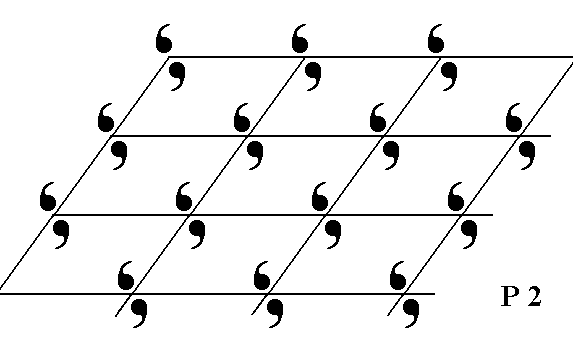```
``````
```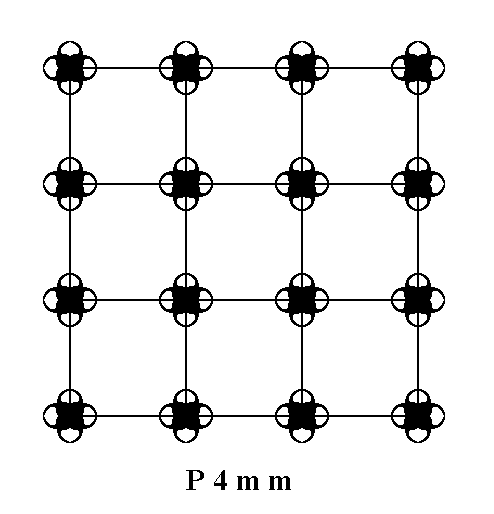```
``````
``````
``````
``````
``````
```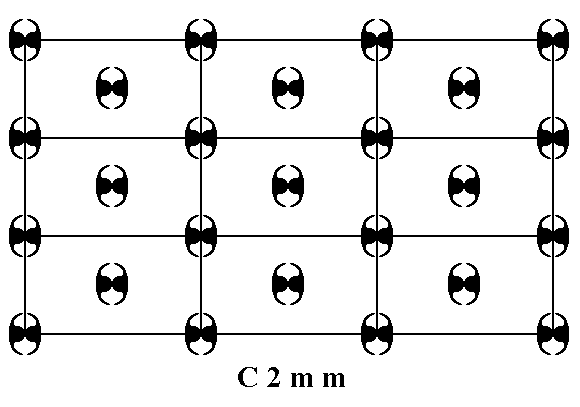```
``````
``````
``````
``````
```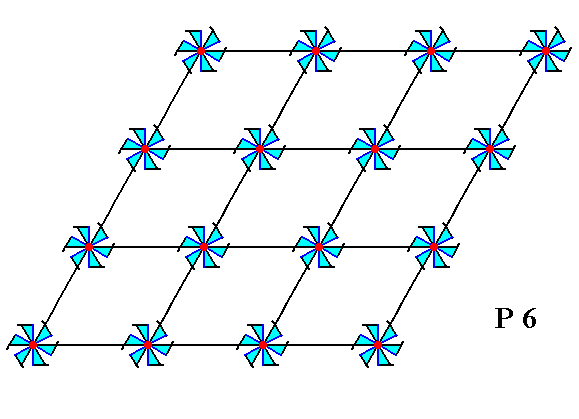```
``````
```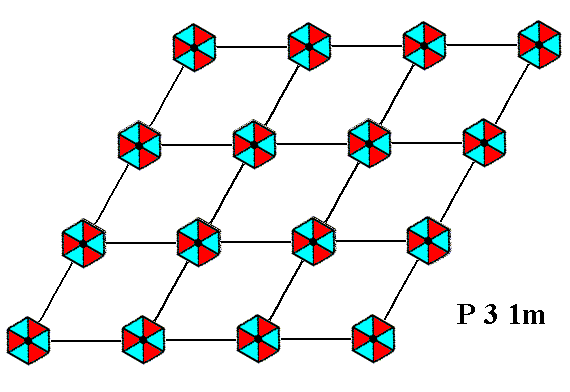```
``````
```
This concludes our summary of the 17 Plane Groups (and implicitly of the 10 planar Point Groups) referring to two-dimensional periodic patterns.

In the next Part we will utilize the knowledge we've gained so far, in order to begin to understand the development of 'faces' (and with it the shapes) of 2-D crystals. All this is necessary to finally understand three-dimensional shapes of natural inorganic beings (3-D crystals).

```

```
```